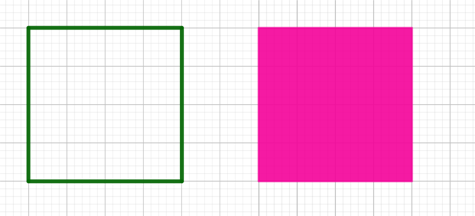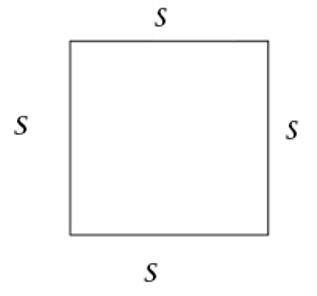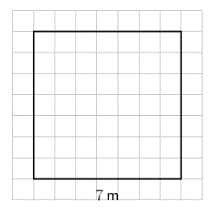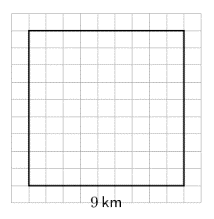Home Practice
For learners and parents For teachers and schools
Textbooks
Full catalogue
Pricing SupportLog in

We think you are located in United States. Is this correct?

# Area and perimeter of squares

## 16.3 Area and perimeter of squares

Perimeter is the total distance around the outside of a shape.

Area is the total space or surface of a flat shape.

perimeter
the total distance around the outside of a shape
area
the total space or surface of a flat shape

In the diagram below, the green line shows the perimeter of the square and the pink surface shows the area of the square.The perimeter of a square is equal to the sum of the lengths of its four sides. We know that all sides of a square are equal in length, so the perimeter is equal to four times the length of the square ($$s$$).$\text{Perimeter of a square } = s + s + s + s = 4 \times s = 4s$

The area of a square is equal to the length of a side squared:

$\text{Area of a square } = s \times s = s^2$

## Worked example 16.6: Calculating the perimeter of a square

Determine the perimeter of the square.### Calculate the perimeter of the square.

To determine the perimeter, we must find the sum of all four sides:

$\text{Perimeter of the square } = 7 + 7 + 7 + 7 = 4 \times 7 = 28 \text{ m}$

$\text{Perimeter of the square} = 28 \text{ m}$

Hint: Always remember to include the units in the answer.

## Worked example 16.7: Calculating the area of a square

Find the area of the square.### Calculate the area of the square.

$\text{Area of the square } = 9 \times 9 = 9^2 = 81 \text{ km}^2$

$\text{Area of the square} = 81 \text{ km}^2$